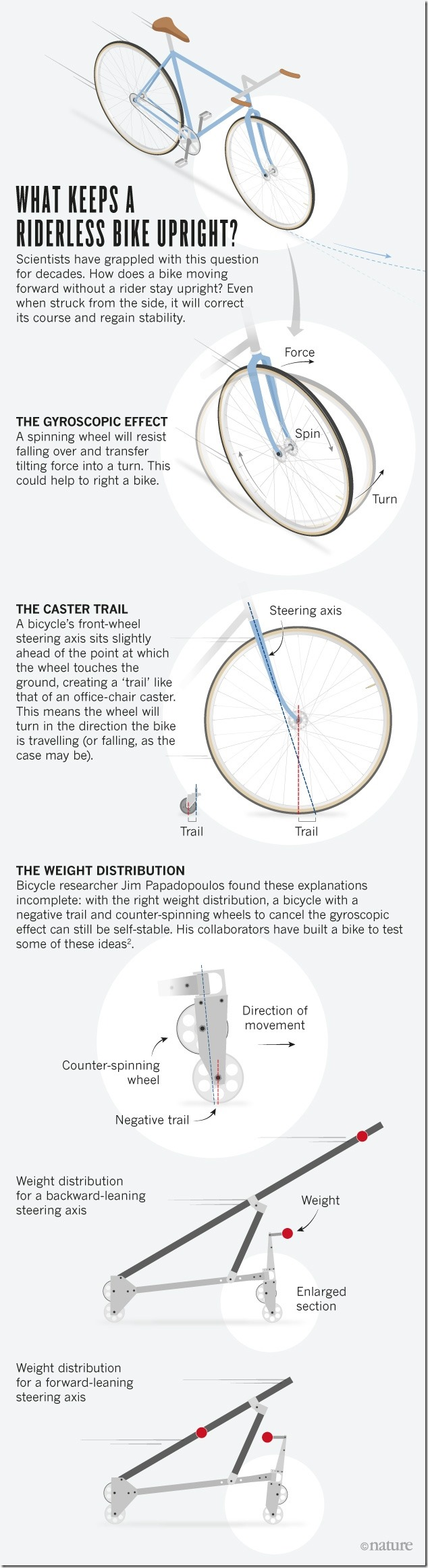Categories

## The Math of Bikes in Motion

Fascinating read in Nature Magazine published July 2016Categories

## How Did Sherlock Know Which Way The Bike Was Headed?

And while this is very interesting, I do wonder about the rake implications and how I can determine bike handling characteristics based on it.  I will ponder that and look for a mathematical answer, perhaps from differential geometry.Mathematical Impressions: Bicycle Tracks

A nice mathematical puzzle, with a solution anyone can understand, is to determine the direction a bicycle went when you come upon its tracks. The answer involves thinking about tangent lines, geometric constraints and the bicycle’s steering mechanism.

https://www.simonsfoundation.org/multimedia/mathematical-impressions-bicycle-tracks/